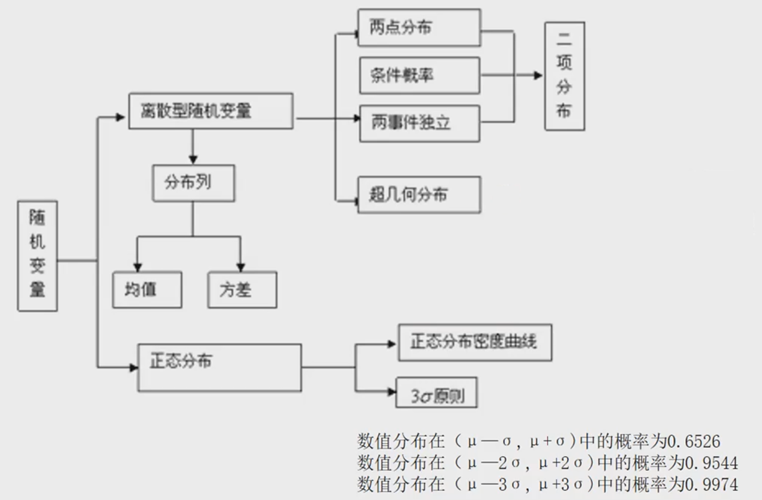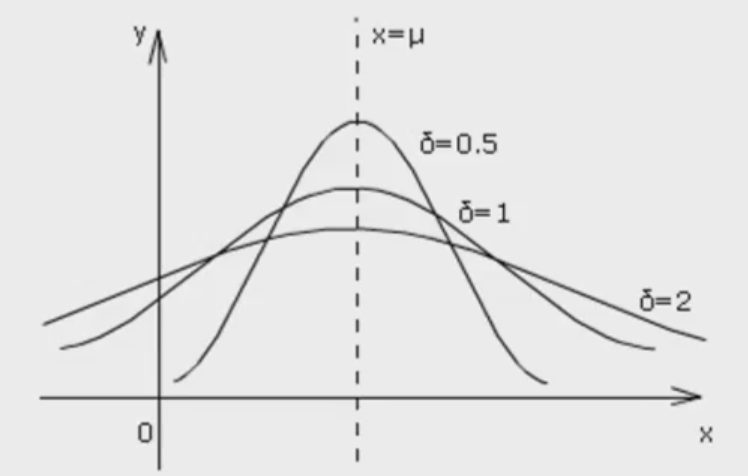# 高中数学基础：随机变量及其分布

## 基本概念## 离散型随机变量

（1）随机变量：如果随机试验的结果可以用一个变量来表示，那么这样的变量叫做随机变量。随机变量常用字母$X,Y,\xi,\eta$ 等表示。

（2）离散型随机变量：对于随机变量可能取的值，可以按一定次序一一列出，这样的随机变量叫做离散型随机变量。

（3）连续型随机变量：对于随机变量可能取的值，可以取某一区间内的一切值，这样的变量就叫做连续型随机变量。

（4）离散型随机变量与连续型随机变量的区别与联系：离散型随机变量与连续型随机变量都是用变量表示随机试验的结果；但是离散型随机变量的结果可以按一定次序一一列出，而连续型随机变量的结果不可以一一列出。

（5）若$X$是随机变量，$Y=aX+b$（$a,b$是常数），则$Y$也是随机变量，并且不改变其属性（离散型、连续型）。

## 离散型随机变量的分布列

### 概率分布（分布列）

$X$ $x_1$ $x_2$ $···$ $x_i$ $···$ $x_n$
$P$ $p_1$ $p_2$ $···$ $p_i$ $···$ $p_n$

① $p_i≥0,i=1,2,…n$
② $\sum_{i=1}^np_i=1$

### 两点分布

$X$ 0 1
$P$ $1-p$ $p$

### 二项分布

$P(X=k) = C_n^kp^k(1-p)^{n-k}$，其中$k=0,1,2,…,n, , q=1-p$，于是得到随机变量$X$的概率分布如下：

$X$ 0 1 $···$ $k$ $···$ $n$
$P$ $C_n^0p^0q^n$ $C_n^1p^1q^{n-1}$ $···$ $C_n^kp^kq^{n-k}$ $···$ $C_n^np^nq^0$

① 独立性：即一次试验中事件发生与否二者必居其一；
② 重复性：即试验是独立重复地进行了$n$次
③ 等概率性：在每次试验中事件发生的概率均相等。

### 离散型随机变量的均值与方差

（1）离散型随机变量的均值

$X$ $x_1$ $x_2$ $···$ $x_i$ $···$ $x_n$
$P$ $p_1$ $p_2$ $···$ $p_i$ $···$ $p_n$

$E(X)=x_1p_1+x_2p_2+···+x_ip_i+···+x_np_n$

① $E(aX+b)=aE(X)+b$
② 若$X$服从两点分布，则$E(X)=p$
③ 若$X~B(n,p)$，则$E(X)=np$

（2）离散型随机变量的方差

$X$ $x_1$ $x_2$ $···$ $x_i$ $···$ $x_n$
$P$ $p_1$ $p_2$ $···$ $p_i$ $···$ $p_n$

$D(X)=\sum_{i=1}^n(x_i-E(X))^2p_i$

① $D(aX+b)=a^2D(X)$
② 若$X$服从两点分布，则$D(X)=p(1-p)$
③ 若$X~B(n,p)$，则$D(X)=np(1-p)$

## 正态分布

$$f(x)=\frac{1}{\sqrt{2\pi}·\sigma}e^{-\frac{(x-\mu)^2}{2\sigma^2}}, , x \in R$$